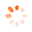Home / Products

# DESCRIPTION

Technical Description

During deceleration, acceleration and deflection of a flowing fluid, there is a change of velocity and thus a change in momentum. Changes in momentum result in forces. In practice, the motive forces are used to convert kinetic energy into work done, for example in a Pelton turbine.

In HM 150.08 jet forces are generated and studied with the aid of a water jet that acts on and is diverted by an interchangeable deflector.

The experimental unit includes a transparent tank, a nozzle, four interchangeable deflectors with different deflection angles and a weight-loaded scale. The force of the water jet is adjusted via the flow rate.

Experiments study the influence of flow velocity and flow rate as well as of different deflection angles. The jet forces generated by the water jet are measured on the weight-loaded scale. The forces are calculated using the momentum equation and compared with the measurements.

The experimental unit is positioned easily and securely on the work surface of the HM 150 base module. The water is supplied and the flow rate measured by HM 150. Alternatively, the experimental unit can be operated by the laboratory supply.

The well-structured instructional material sets out the fundamentals and provides a step-by-step guide through the experiments.Learning Objectives / Experiments

- demonstration of the principle of linear momentum
- study of the jet forces
- influence of flow rate and flow velocity
- influence of different deflection angles

# Subscribe now